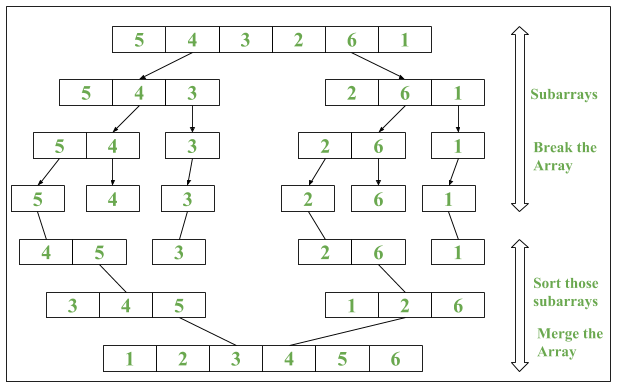Related Articles

# Java 8 | Arrays parallelSort() method with Examples

• Difficulty Level : Easy
• Last Updated : 11 Dec, 2018

Java 8 introduced a new method called as parallelSort() in java.util.Arrays Class. It uses Parallel Sorting of array elements

Algorithm of parallelSort()

```1. The array is divided into sub-arrays and that
sub-arrays is again divided into their sub-arrays,
until the minimum level of detail in a set of array.
2. Arrays are sorted individually by multiple thread.
3. The parallel sort uses Fork/Join Concept for sorting.
4. Sorted sub-arrays are then merged.```

Syntax :

1. For sorting data in ascending order :
```public static void parallelSort(Object obj[])

For sorting data in specified range in ascending order :public static void parallelSort(Object obj[], int from, int to)```

parallelSort() method uses concept of MultiThreading which makes the sorting faster as compared to normal sorting method.

ExampleBelow are the program that will illustrate the use of Arrays.parallelSort():

Program 1: To demonstrate use of Parallel Sort

 `// Java program to demonstrate``// Arrays.parallelSort() method`` ` `import` `java.util.Arrays;`` ` `public` `class` `ParallelSort {``    ``public` `static` `void` `main(String[] args)``    ``{``        ``// Creating an array``        ``int` `numbers[] = { ``9``, ``8``, ``7``, ``6``, ``3``, ``1` `};`` ` `        ``// Printing unsorted Array``        ``System.out.print(``"Unsorted Array: "``);``        ``// Iterating the Elements using stream``        ``Arrays.stream(numbers)``            ``.forEach(n -> System.out.print(n + ``" "``));``        ``System.out.println();`` ` `        ``// Using Arrays.parallelSort()``        ``Arrays.parallelSort(numbers);`` ` `        ``// Printing sorted Array``        ``System.out.print(``"Sorted Array: "``);``        ``// Iterating the Elements using stream``        ``Arrays.stream(numbers)``            ``.forEach(n -> System.out.print(n + ``" "``));``    ``}``}`
Output:
```Unsorted Array: 9 8 7 6 3 1
Sorted Array: 1 3 6 7 8 9
```

Time Complexity is O(nlogn)

Program 2: To demonstrate use of Parallel Sort w.r.t. Series Sort (Normal Sort)

 `// Java program to demonstrate impact ``// of Parallel Sort vs Serial Sort `` ` `import` `java.util.Arrays; ``import` `java.util.Random; `` ` `public` `class` `ParallelSort { ``    ``public` `static` `void` `main(String[] args) ``    ``{ ``        ``// Creating an array ``        ``int` `numbers[] = ``new` `int``[``100``]; `` ` `        ``// Iterating Loop till i = 1000 ``        ``// with interval of 10 ``        ``for` `(``int` `i = ``0``; i < ``1000``; i += ``10``) { `` ` `            ``System.out.println(``"\nFor iteration number: "``                            ``+ (i / ``10` `+ ``1``)); `` ` `            ``// Random Int Array Generation ``            ``Random rand = ``new` `Random(); `` ` `            ``for` `(``int` `j = ``0``; j < ``100``; j++) { ``                ``numbers[j] = rand.nextInt(); ``            ``} `` ` `            ``// Start and End Time of Arrays.sort() ``            ``long` `startTime = System.nanoTime(); `` ` `            ``// Performing Serial Sort ``            ``Arrays.sort(numbers); `` ` `            ``long` `endTime = System.nanoTime(); `` ` `            ``// Printing result of Serial Sort ``            ``System.out.println(``"Start and End Time in Serial (in ns): "``                            ``+ startTime + ``":"` `+ endTime); ``            ``System.out.println(``"Time taken by Serial Sort(in ns): "``                            ``+ (endTime - startTime)); `` ` `            ``// Start and End Time of Arrays.parallelSort() ``            ``startTime = System.nanoTime(); `` ` `            ``// Performing Parallel Sort ``            ``Arrays.parallelSort(numbers); `` ` `            ``endTime = System.nanoTime(); `` ` `            ``// Printing result of Parallel Sort ``            ``System.out.println(``"Start and End Time in parallel (in ns): "``                            ``+ startTime + ``":"` `+ endTime); ``            ``System.out.println(``"Time taken by Parallel Sort(in ns): "``                            ``+ (endTime - startTime)); ``            ``System.out.println(); ``        ``} ``    ``} ``} `
Output:
```For iteration number: 1
Start and End Time in Serial (in ns): 3951000637977:3951000870361
Time taken by Serial Sort(in ns): 232384
Start and End Time in parallel (in ns): 3951000960823:3951000971044
Time taken by Parallel Sort(in ns): 10221

For iteration number: 2
Start and End Time in Serial (in ns): 3951001142284:3951001201757
Time taken by Serial Sort(in ns): 59473
Start and End Time in parallel (in ns): 3951001256643:3951001264039
Time taken by Parallel Sort(in ns): 7396
.
.
.
For iteration number: 99
Start and End Time in Serial (in ns): 3951050723541:3951050731520
Time taken by Serial Sort(in ns): 7979
Start and End Time in parallel (in ns): 3951050754238:3951050756130
Time taken by Parallel Sort(in ns): 1892

For iteration number: 100
Start and End Time in Serial (in ns): 3951050798392:3951050804741
Time taken by Serial Sort(in ns): 6349
Start and End Time in parallel (in ns): 3951050828544:3951050830582
Time taken by Parallel Sort(in ns): 2038
```

Note : Different time intervals will be printed But parallel sort will be done before normal sort.

Environment: 2.6 GHz Intel Core i7, java version 8

Attention reader! Don’t stop learning now. Get hold of all the important DSA concepts with the DSA Self Paced Course at a student-friendly price and become industry ready.  To complete your preparation from learning a language to DS Algo and many more,  please refer Complete Interview Preparation Course.

In case you wish to attend live classes with experts, please refer DSA Live Classes for Working Professionals and Competitive Programming Live for Students.

My Personal Notes arrow_drop_up